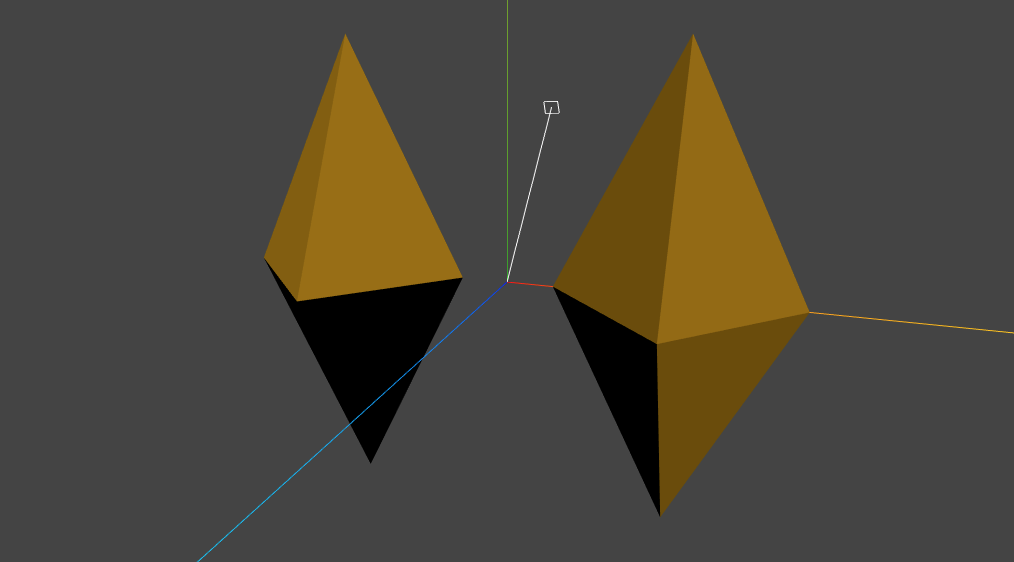# InstancedMesh uses an incorrect normal matrix

As discussed here.

The vertex normal should be transformed by the normal matrix computed from the `instanceMatrix`.

In this image, the mesh on the left is an `InstancedMesh` having a single instance. The image on the right is a non-instanced `Mesh`. They should have identical shading.##### Three.js version
• [ x ] r113 dev
• [ x ] r112

## 1 thought on “InstancedMesh uses an incorrect normal matrix”

1. Anonymous says:

FWIW normal matrix isn’t the only way to transform normals, assuming the object matrix uses rotation and (non-uniform) scale there’s an alternative formulation.

I’m going to use column vectors below, so the vertex transform is `T*R*S*v` with a decomposed matrix. The canonical formulation for normal matrix suggests using `NM = inverse(transpose(R*S))`.

`inverse(transpose(R*S)) = inverse(transpose(S) * transpose(R)) = inverse(transpose(R)) * inverse(transpose(S))`, where R is a rotation matrix and S is a diagonal matrix with scale values for each axis.

`inverse(transpose(R)) = R`, and `transpose(S) = S`, so the above is equal to `R * inverse(S)`, which is equal to `R * S * inverse(S) * inverse(S)`.

Thus it’s sufficient to pre-transform the object-space normal using `inverse(S)^2` – since S is a diagonal, if you know the scale values this just involves dividing the normal by scale^2.

You can recover the scale values from the combined R*S matrix by measuring the length of basis vectors.

This shader code illustrates the construction:

``````vec3 RX = modelViewMatrix.xyz;
vec3 RY = modelViewMatrix.xyz;
vec3 RZ = modelViewMatrix.xyz;

vec3 transformedNormal = objectNormal;
transformedNormal /= vec3(dot(RX, RX), dot(RY, RY), dot(RZ, RZ));
transformedNormal = (modelViewMatrix * vec4(transformedNormal, 0.0)).xyz;
transformedNormal = normalize(transformedNormal);
``````

The cost of this correction is three dot products and a vector division, which seems reasonable. If both instance matrix and object matrix can carry non-uniform scale then I think you will need to run this code twice.

Something like this could be used as a generic normal transform function (assuming a normalization step is ran after this):

``````vec3 transformNormal(vec3 v, mat3 m)
{
return m * (v / vec3(dot(m, m), dot(m, m), dot(m, m)));
}
``````

edit The above assumes that the transform matrix can be decomposed into R*S, which isn’t true of an arbitrary sequence of rotation-scale transforms, but is probably true for instance matrix transform – so I’m assuming this can be combined with using normalMatrix for handling the general scene graph transform. So this might be useful not as a replacement for existing normalMatrix, but purely as a way to correct instanceMatrix transformation in the shader.